# 孔位度综合检测工具设计与使用规范

### 发布日期： 2021-09-24 点击： 113

[摘要] 检具是指零件加工制造过程中生产线上的专用（尺寸）检具（简称检具）。检具既具有定量功能又具有定性功能（用于非加工件的检具也具有测量支撑功能），检具设计检具设计，其测量功能、定位原理应满足技术要求图纸测量，保证加工制造；测量评价基准的一致性。综合检具适用于汽车零部件等量产产品，用于更换卡尺、塞规、CMM（三坐标测量机）、游标卡尺等量具。操作简单，使用方便、省时、省力、可靠。，检查效率高。本文设计的检具主要是指用于测量各加工孔位置的计数型（定性功能）综合检具。它用于检查最大实体所需的测量元素和（或）参考元素，以确定其实际轮廓。是否超出相应的边界。它用于检查最大实体所需的测量元素和（或）参考元素，以确定其实际轮廓。是否超出相应的边界。它用于检查最大实体所需的测量元素和（或）参考元素，以确定其实际轮廓。是否超出相应的边界。

[关键词] 孔位设计组合检查工具

1 孔位综合检测工具设计

1.1 检具定位装置的设计

1.1.1 定位销B的定位部分尺寸

DMV=DM-t=(6.09-0.02）-0.05=6.02mm

Tt=TD+t=(0.02+0.02）+0.05=90μm;

TP=4μm；Fp=12μm；WP=4μm；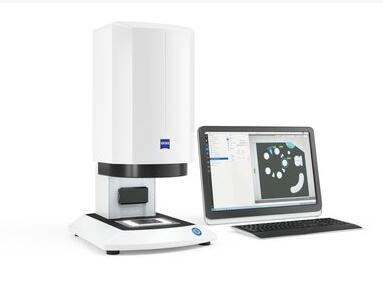dBP(B)=DMV

dLP(B)=(dBP(B)+Fp(B))0-TP=(6.02+0.012）0-0.004

=6.0320-0.004mm

dwP(B)=(dBP(B)+Fp(B))-(TP+WP)=(6.02+0.012）-(0.004 +0.004）=6.024mm

1.1.2 定位销C的定位部分尺寸

DMV=DM-t=(6.09-0.02）-0.10=5.97mm

Tt=TD+t=(0.02+0.02）+0.10=140μm;

TP=5μm；Fp=16μm；WP=5μm；

dBP(C)=DMV

dLP(C)=(dBP(C)+Fp(C))0-TP=(5.97+0.016）0-0.005=5.9860-0.005mm

dwP(C)=(dBP(C)+Fp(C))-(TP+WP)=(5.97+0.016）-(0.005 +0.005）=5.976mm（如图3）

1.2 确定测量部分的尺寸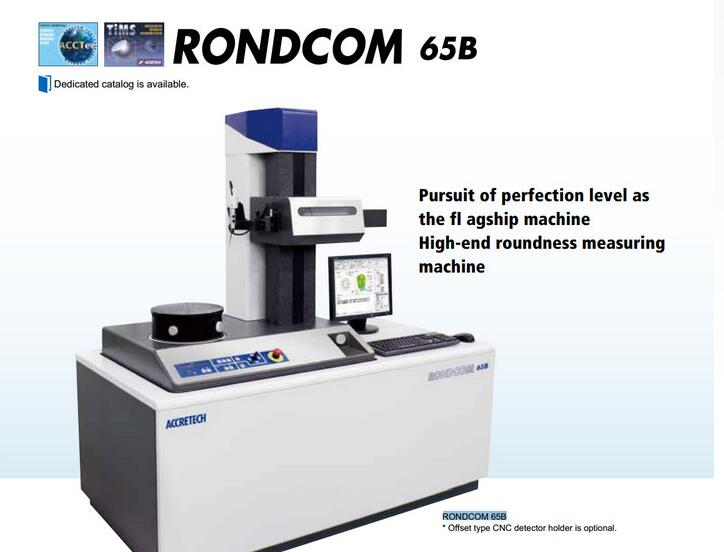Tt=(0.05+0.05）+0.5=0.60mm

DMMC=12-0.05=11.95mm

d1BM=DMMC-t=11.95-0.5=11.45mm

d1LM=(d1BM+FM1）0-TM=(11.45+0.045）0-0.01=11. 4950-0.01mm

d1WM=(d1BM+FM1）-(TM+WM)=(11.45+0.045）-(0.01+0.01）=11.475

1.3 确定导向部分的尺寸

DBG=dLMmax=11.495mm

DLG=(DBG+Cmin)+TG0=(11.495+0.005）+0.0060=11.5+0.@ >0060mm

DWG=(DBG+Cmin)+(TG+WG)=(11.495+0.005）+(0.006+ 0.00< @6）

=11.512mm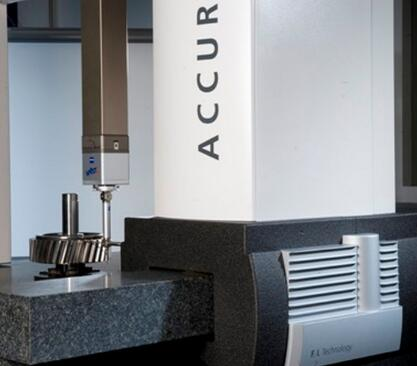DMMC——孔的最大尺寸

t—中心元素的几何公差值

dBP、dLP、dwP——基本尺寸、限制尺寸、

dBM、dLM、dwM——测量部分的基本尺寸和极限标尺

DBG、DLG、DWG——导向件的基本尺寸和限位尺

Tt——综合公差

tP——工作部位的位置公差

TM、TP、TG——测量件、定位件和导向件的尺寸公差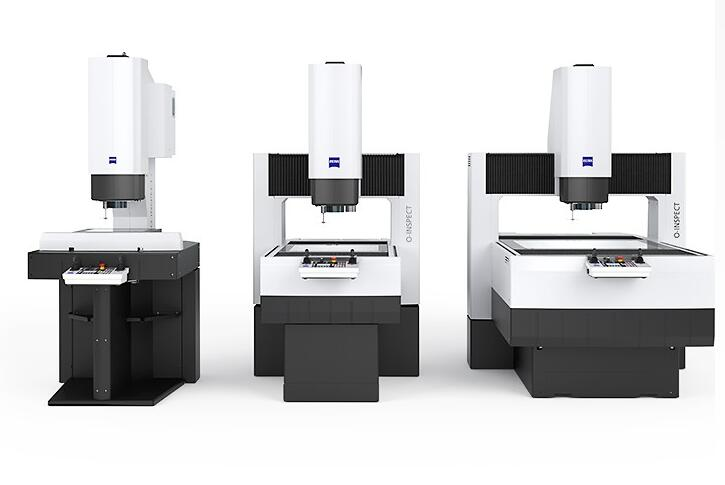WM、WP、WG——测量部分、定位部分和导向部分的最小允许磨损

Cmin——导向部分的最小间隙

FM、FP——测量站点和定位站点的基础

TM、TP、TG、WM、WP、WG、Cmin、tP的值按GB8069-87表2求出。

1.4 夹紧装置设计

T=K*F0*d=0.21*2000max（N)*6*10-3m=2.52N·m.（max）

d——螺纹公称直径，mm；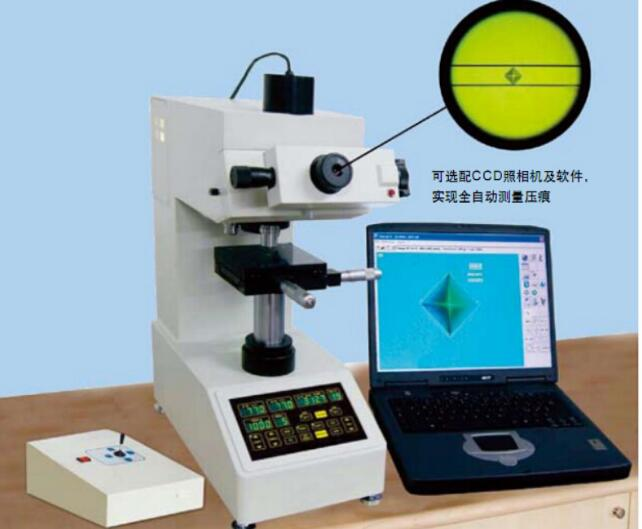F0——预紧力，N；

K——拧紧扭矩系数；

K的值根据《机械设计手册》第4章连接与紧固，第1章螺纹与螺纹连接3.3.1螺纹连接紧固扭矩计算表4-1-60取< @0.21。

1.5 主要技术要求

2 综合定位检测工具的使用

3 结论

 程大贤．连接和紧固。机械设计手册。小册子。北京：化学工业出版社，2004.1.

 国家机械工业委员会．位置规，GB8069-871987-07-14.

 陈玉平，高小康．互换性和测量技术，（第二版）。高等教育出版社，2005.7.

### 本文网址：www.ks-wbt.vip/industry/4456.html

•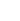在线客服
•微信二维码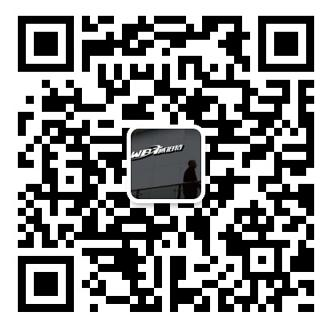•在线留言
•